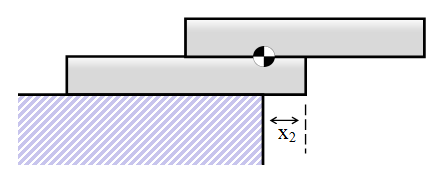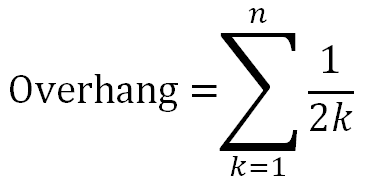# How to pile up stuff

Hi,
today I will write about block (in my case) or also book stacking problem. This is fascinating problem and I want you to try to take twenty cards or same blocks. Your quest is to stack them on top of each other but at the same time try to hang them over side of table as much as you can.

It should look something like this:How much “overhang” can you theoreticly do? With twenty cards the overhand will be maximally 1.79886982857. You can only put one card on another of course.

What is this thing anyway?

Well there is nice physics and math involved behind!

Lets start with the proof why you can actually do this in the first place. Try to ask someone around “How much is it possible to overhang infinte amount of blocks?” most people will probably tell you that the answer is 1/2 which is kind of intuitive, but not true as you already know if you tried the experiment that I told you to do in the beginning.

It all has to do with the center of mass. This is the place that you are trying to balance at the edge of table. As soon as the center is moved behind the edge it will fall.

For first card it is easy, you can truly get overhang of only 1/2 of the width of your block.

For two objects you can get better, actually 3/4! The first block (highest) will stay the same, it will be exactly at half of the previous one to maximize the effect. For this the center of mass will be on the edge. What about the one under that? Well there comes the problem because it combines center of mass with the one above which means that it cannot stretch so far. It carries two times the mass, lets say 2MFirst block goes like this: X*M where M is the mass and X is the distance it can stretch, it is 1/2. This must equal also for the second block which carries two so there are two “masses”.

X*2M=X*M (X is different value every time)

X*2M=1/2M (because as I said X for first block is 1/2)

2X=1/2 (divides by M)

X=1/4

the second block has to be one fourth of its width from the table.

For third one — X*3M=1/2M …. X=1/6

Fourth = 1/8

As you continue you will find that this goes in particular series called “harmonic series”.

1/2 + 1/4 + 1/6 + 1/8…

This series sums up to infinity so you can theoreticly make any overhang you want!

For the overhang of 1 you need only four — 1/2+1/4+1/6+1/8=25/24=1.04166For overhang two you need 31!
For three you need 227!
For four you need 1,674!
For five you need 12,367!
For six you need 91,381!
For ten you need 272,400,601!

As you can see it increases pretty rapidly.Now just enjoy me trying to get to the best possible overhang:

Dragallur

 Harmonic series are just 2x bigger: 1+ 1/2 + 1/4 + 1/6 + 1/8…

I used these pages as resources both for pictures and for information: 1) 2) (only first picture)